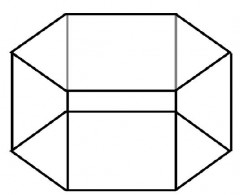# How Many Faces Does A Hexagonal Prism Have?

Hi I am Danian,I know for sure that a hexagonal has 8 faces,12vertices and18 edges
thanked the writer.
It haves 8 faces and 18 edges and 12 vertices
thanked the writer.
It is a prism which has a hexagonal base so it is called hexagonal prism. Hexagonal prism has eight faces. It has eighteen edges and twelve vertices. The volume of the hexagonal prism can be calculated by the formula;

V= 33/2/2 a2* h

a= length of the side

h= height of hexagonal prism
thanked the writer.
It has 8 faces
thanked the writer.
8 I'm shore
thanked the writer.
8...smart one
thanked the writer.
8
short and sweet
thanked the writer.None! A hexagonal prism has:

• Two hexagonal faces
• Six rectangular faces
thanked the writer.
I think 8
thanked the writer.
6,7 or 8.
thanked the writer.
It has 7 faces.
thanked the writer.